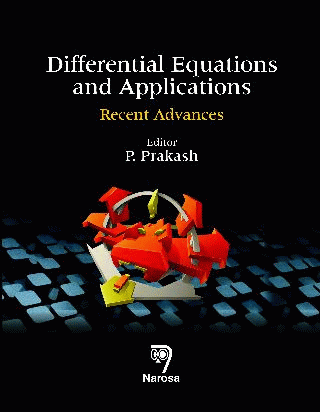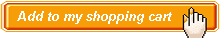Differential Equations and Applications:Recent Advances Authors:   P. Prakash ISBN: 978-81-8487-331-3 Publication Year:   2014 Pages:   242 Binding:   Hard Back About the book DIFFERENTIAL EQUATIONS AND APPLICATIONS: Recent Advances focus on the latest developments in Nonlinear Dynamical Systems, Neural Networks, Fluid Dynamics, Fractional Differential Systems, Mathematical Modelling and Qualitative Theory. Different aspects such as Existence, Stability, Controllability, Viscosity and Numerical Analysis for different systems have been discussed in this book. This book will be of great interest and use to researchers in Applied Mathematics, Engineering and Mathematical Physics. Key Features Table of content Analytical and numerical solutions of an one dimensional fractional sub-diffusion equation with Neumann boundary conditions / Inverse problems for reaction diffusion system / Existence and controllability results for fractional dynamical systems / Relative controllability of stochastic impulsive systems with delays in control / A priori error estimates of mixed finite element methods for an optimal control problem governed by integro-differential equations / An analytic solution of a viscoelastic fluid over an impermeable stretching sheet with non-uniform heat source/sink, elastic deformation and radiation effect / Differential transform method for solving particular third order linear and nonlinear ordinary differential equations / Proton dynamics in polypeptide chains governed by higher order Nonlinear Schrödinger equation / Novel periodic solutions of a Boussinesq equation / Exact solitary solutions of integrable (2 + 1) dimensional generalized Sasa-Satsuma equation / New exact travelling wave solutions to the nonlinear evolution equation governing the spin dynamics using symbolic computation / Existence of periodic solitary wave solutions in a nematic liquid crystal system / Existence results for impulsive neutral functional integro-differential equations in Banach space / Oscillation of neutral hyperbolic differential equation with deviating arguments and damping term / Oscillatory behavior of third order neutral differential equation with mixed nonlinearities / Existence of p-th moment almost automorphic to a class of non-autonomous stochastic fractional differential equations / Unsteady viscous flow through a porous channel with injection and suction / Diffusion-driven instability and bifurcation analysis for a Holling-Tanner predator-prey model / Mobile kinkon solutions of a NLPDE governing the dynamics of nematic liquid crystal using Exp-function method / Approximate analytic solutions and exact numerical solutions of (ut + uux – ?uxx)x + uyy = 0 / Robust stability of neutral systems with Takagi-Sugeno (T-S) fuzzy model and interval time-varying delays / Robust asymptotic stability criteria for Takagi-Sugeno fuzzy neural networks with mixed interval time-varying delays / Existence of mobile and stair solitons of (3 + 1)-dimensional generalized Davey-Stewartson equations using Exp-function method / Exponential stability for fuzzy neural networks with impulses: A delay fractioning approach / New relaxed LMI conditions of stochastic recurrent neural networks with time varying delays and polytopic uncertainties / Painlevé analysis, Bäcklund and Cole - Hopf transformation of the (2 + 1) and (3 + 1)-dimensional Burgers equations.Audience Postgraduate Students, Libraries, Researchers and Professionals